# Get these flashcards, study & pass exams. For free! Even on iPhone/Android!

## Basic Trigonometric Identities & Laws (29 Cards)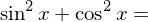1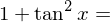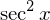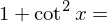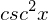What is the sine rule?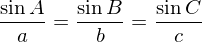What is the cosine rule ?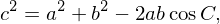Sin (A+B)=
SinACosB+CosASinB
Cos(A+B)=
CosACosB-SinASinB
Tan(A+B)=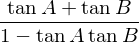Sin (A-B)
SinACosB-CosASinB
Cos (A-B)
CosACosB+SinASinB
Tan (A-B)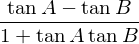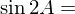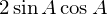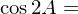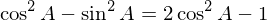Tan (2A)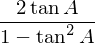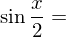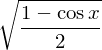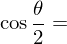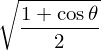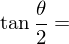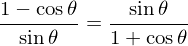sin ( π / 2 – x )
cos x
cos ( π / 2 – x )
sin x
tan ( π / 2 – x )
cot x
sin ( π – x )
sin x
cos ( π – x )
-cos x
tan ( π – x )
-tan x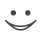Flashcard set info:
Author: CoboCards-User
Main topic: Mathematics
Topic: Trigonometry
Published: 23.07.2013

Card tags:
All cards (29)
no tagsReport abuse